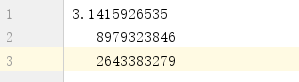一、从文件中读取数据

pi.txt1.读取整个文件

``````with open('pi.txt') as file_object:
print(contents)``````

2.逐行读取

``````with open('pi.txt') as file_object:
for line in file_object:
print(line.rstrip())``````

3.创建一个包含文件各行内容的列表

``````with open('pi.txt') as file_object:

for line in lines:
print(line.rstrip())``````

二、写入文件

1.写入空文件

``````filename = 'programming.txt'

with open(filename, 'w') as file_object:
file_object.write("I Love Programming!")``````

2.写入多行

3.附加到文件

三、存储数据

number_write.py

``````import json

numbers = [2, 3, 4, 5, 6]

filename = 'number.json'
with open(filename, 'w') as f_obj:
json.dump(numbers, f_obj)``````

``````import json

filename = 'number.json'
with open(filename) as f_obj:

print(numbers)``````

0条评论